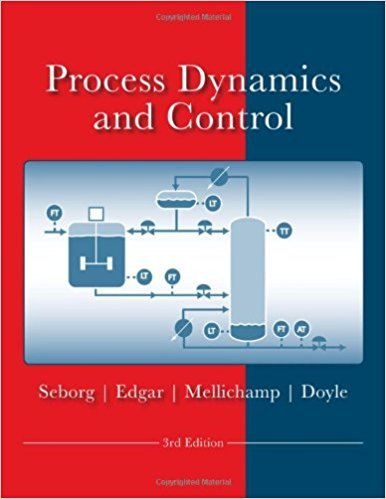×
Get Full Access to Process Dynamics And Control - 3 Edition - Chapter 3 - Problem 3.5
Get Full Access to Process Dynamics And Control - 3 Edition - Chapter 3 - Problem 3.5

×

# The start-up procedure for a batch reactor includes aISBN: 9780470128671 148

## Solution for problem 3.5 Chapter 3

Process Dynamics and Control | 3rd Edition

• Textbook Solutions
• 2901 Step-by-step solutions solved by professors and subject experts
• Get 24/7 help from StudySoup virtual teaching assistantsProcess Dynamics and Control | 3rd Edition

4 5 1 342 Reviews
12
2
Problem 3.5

The start-up procedure for a batch reactor includes a heating step where the reactor temperature is gradually heated to the nominal operating temperature of 75C. The desired temperature profile T(t) is shown in Fig. E3.5. What is T(s)?

Step-by-Step Solution:

Problem 3.5The start-up procedure for a batch reactor includes a heating step where the reactortemperature is gradually heated to the nominal operating temperature of 75°C. The desiredtemperature profile T(t) is shown in Fig. E3.5. What is T(s) Step-by-step solution Step 1 of 3 ^Refer to Figure E3.5 in the text book; consider the following points to develop a desiredtemperature profile T(t).At t = 0, height of the step is 20. So, the desired temperature profile for t = 0 is 20S(t).At t = 30 min, there is an increase in slope of the function; so add a ramp with a slope of(rise/run) = (75 - 20/30). Thus, the desired temperature profile for t = 30 min istS(t). 30At t > 30 min, the temperature profile becomes constant. So, the slope of this function is 55 30 (t - 30)S(t - 30).

Step 2 of 3

Step 3 of 3

##### ISBN: 9780470128671

Since the solution to 3.5 from 3 chapter was answered, more than 500 students have viewed the full step-by-step answer. The answer to “The start-up procedure for a batch reactor includes a heating step where the reactor temperature is gradually heated to the nominal operating temperature of 75C. The desired temperature profile T(t) is shown in Fig. E3.5. What is T(s)?” is broken down into a number of easy to follow steps, and 38 words. The full step-by-step solution to problem: 3.5 from chapter: 3 was answered by , our top Engineering and Tech solution expert on 11/15/17, 04:03PM. Process Dynamics and Control was written by and is associated to the ISBN: 9780470128671. This textbook survival guide was created for the textbook: Process Dynamics and Control, edition: 3. This full solution covers the following key subjects: temperature, reactor, Operating, gradually, heated. This expansive textbook survival guide covers 24 chapters, and 420 solutions.

Unlock Textbook Solution